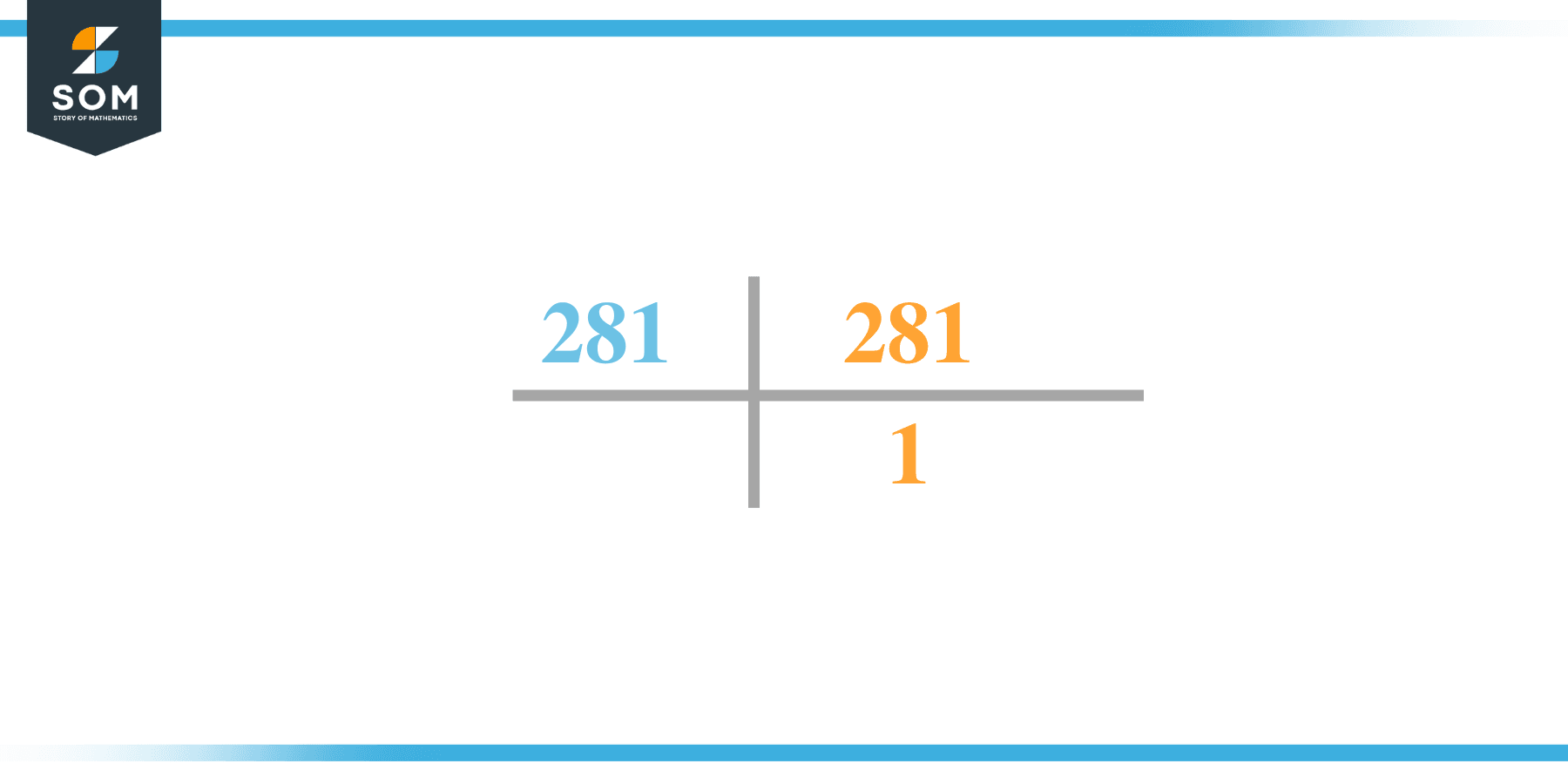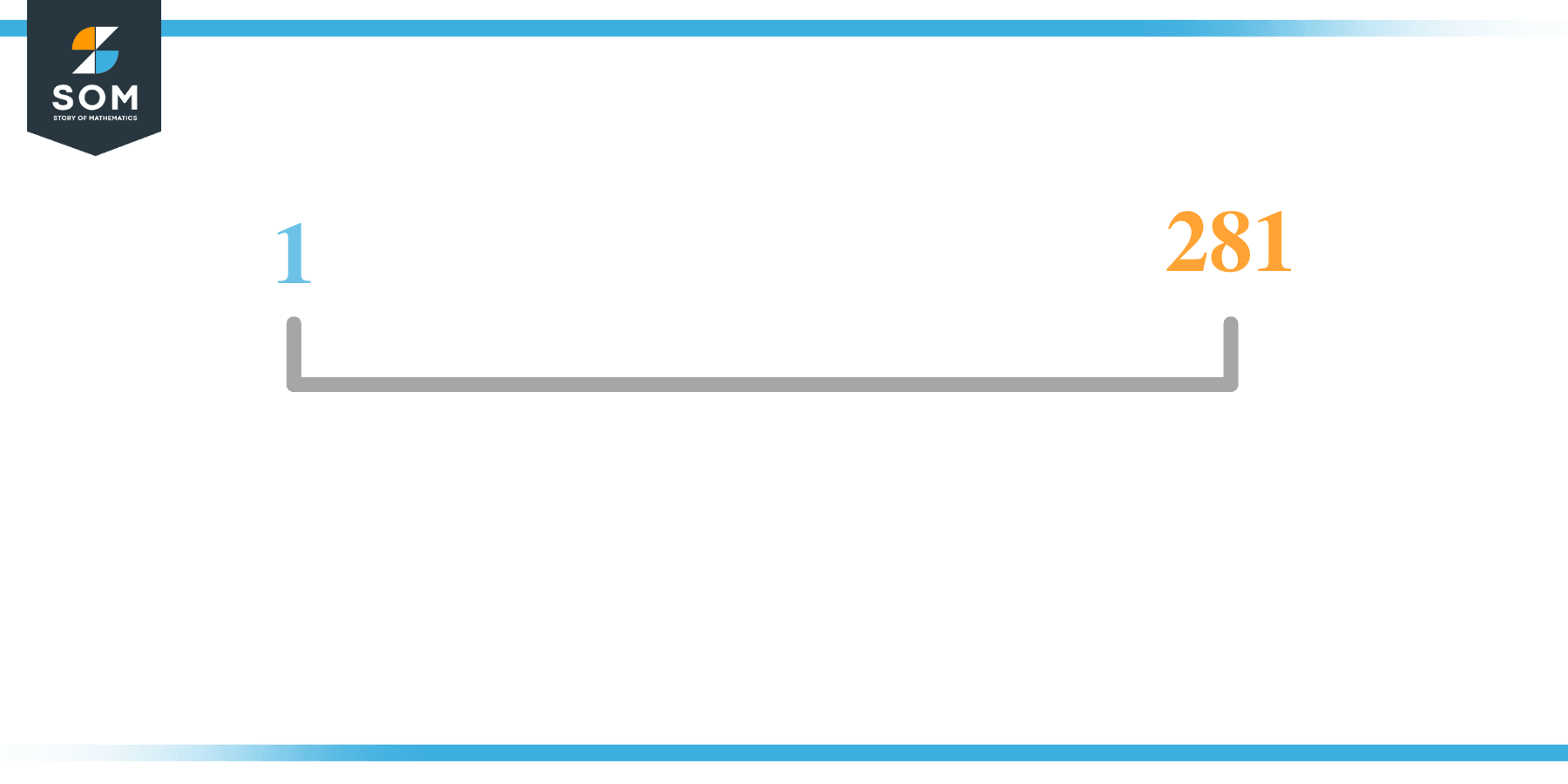# Factors of 281: Prime Factorization, Methods, and Examples

The numbers that are entirely divisible by 281 are termed the factors of 281. Generally, factors of any number are the natural numbers that divide that number evenly with zero remainders.281 is a prime factor with only two factors, i.e., 1 and 281.

### Factors of 281

Here are the factors of number 281.

Factors of 281: 1 and 281

### Negative Factors of 281

The negative factors of 281 are similar to its positive factors, just with a negative sign.

Negative Factors of 28: -1 and -281

### Prime Factorization of 281

The prime factorization of 281 is the way of expressing its prime factors in the product form.

Prime Factorization: 1 x 281

In this article, we will learn about the factors of 281 and how to find them using various techniques such as upside-down division, prime factorization, and factor tree.

## What Are the Factors of 281?

The factors of 281 are 1 and 181. These numbers are the factors as they do not leave any remainder when divided by 281.

The factors of 281 are classified as prime numbers and composite numbers. The prime factors of the number 281 can be determined using the prime factorization technique.

## How To Find the Factors of 281?

You can find the factors of 281 by using the rules of divisibility. The divisibility rule states that any number, when divided by any other natural number, is said to be divisible by the number if the quotient is the whole number and the resulting remainder is zero.

To find the factors of 281, create a list containing the numbers divisible by 281 with zero remainders. One important thing to note is that one and 281 are the 281’s factors, as every natural number has one and the number itself as its factor.

One is also called the universal factor of every number. The factors of 281 are determined as follows:

$\dfrac{281}{1} = 281$

$\dfrac{281}{281} = 1$

Therefore, 1 and 281 are the factors of 281.

### Total Number of Factors of 281

For 281, there are two positive factors and two negative ones. So in total, there are four factors of 281.

To find the total number of factors of the given number, follow the procedure mentioned below:

1. Find the factorization/prime factorization of the given number.
2. Demonstrate the prime factorization of the number in the exponent form.
3. Add 1 to each of the exponents of the prime factor.
4. Now, multiply the resulting exponents together. This obtained product is equivalent to the total number of factors of the given number.

By following this procedure, the total number of factors of X is given as:

Factorization of 281 is 1 x 281.

The exponent of 1 and 281 is 1.

Adding 1 to each and multiplying them together results in 4.

Therefore, the total number of factors of 281 is 4. Two factors are positive, and two factors are negative.

### Important Notes

Here are some essential points that must be considered while finding the factors of any given number:

• The factor of any given number must be a whole number.
• The number factors cannot be in the form of decimals or fractions.
• Factors can be positive as well as negative.
• Negative factors are the additive inverse of the positive factors of a given number.
• The factor of a number cannot be greater than that number.
• Every even number has 2 as its prime factor, the smallest prime factor.

## Factors of 281 by Prime FactorizationThe number 281 is a composite. Prime factorization is a useful technique for finding the number’s prime factors and expressing the number as the product of its prime factors.

Before finding the factors of 281 using prime factorization, let us find out what prime factors are. Prime factors are the factors of any given number that are only divisible by 1 and themselves.

To start the prime factorization of 281, divide by its smallest prime factor. First, determine that the given number is either even or odd. If it is an even number, then 2 will be the smallest prime factor.

Continue splitting the quotient obtained until 1 is received as the quotient. The prime factorization of 281 can be expressed as:

281 = 1 x 281

## Factors of 281 in PairsThe factor pairs are the duplet of numbers that when multiplied together, result in the factorized number. Factor pairs can be more than one depending on the total number of factors.

For 281, the factor pairs can be found as:

1 x 281 = 281

The possible factor pair of 281 is given as (1, 281).

All these numbers in pairs, when multiplied, give 281 as the product.

The negative factor pair of 281 is given as:

-1 x -281 = 281

It is important to note that in negative factor pairs, the minus sign has been multiplied by the minus sign, due to which the resulting product is the original positive number. Therefore, -1 and -281 are called negative factors of 281.

The list of all the factors of 281, including positive as well as negative numbers, is given below.

Factor list of 281: 1, -1, 281, and -281

## Factors of 281 Solved Examples

To better understand the concept of factors, let’s solve some examples.

### Example 1

How many factors of 281 are there?

### Solution

The total number of Factors of 281 is 2.

Factors of 281 are 1 and 281.

### Example 2

Find the factors of 281 using prime factorization.

### Solution

The prime factorization of 281 is given as:

$281 \div 1 = 281$

So the prime factorization of 281 can be written as:

1 x 281 = 281